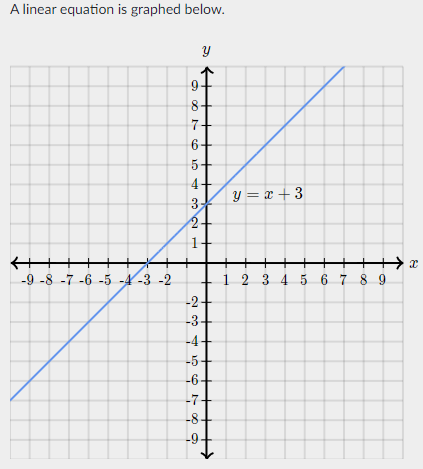mycollegehive
Which of the following represents all solutions (x,y) to th...
SAT-math-test SAT math0Which of the following represents all solutions (x,y) to the system of equations created by the linear equation and the quadratic equation y = -x2 + 9 ?

A. (3, 0) and (-3, 0)

B. (0, 3) and (0, -3)

C. (2, 5) and (-3, 0)

D. (-2, 1) and (3, 6)

90 viewsShareFollowSchool not set Nigeria
19 November 2020University of Lagos Nigeria
20 November 20200Given y = x + 3 and y = -x2 + 9 as the linear and quadratic equation respectively.

Since both equations are represented in the same cordinates (x and y), their solution(s) is simply the pioint where both equations intersect.

Thus,

x + 3 = - x2 + 9

rearrange the above equation

x2 + x - 6 = 0

The above is a quadratic equation and can be simplified by factorization

x2 -2x + 3x - 6 = 0

(x2 -2x) + (3x - 6) = 0

x(x -2) + 3(x - 2) = 0

(x + 3) (x - 2) = 0

x + 3 = 0 and x - 2 = 0

x = -3 and x = 2

To get the y-cordinate, we substitute the values of x into one of the two equations.

Substituting into the linear equation yields:

y = -3 + 3 and y = 2 + 3

y = 0 and y = 5

Therefore, the solutions are:

(2, 5) and (-3, 0)Share

### Groups

How to insert math formulas/equations### Related Tags

SAT-math-test

6 followers

476 questionsSAT

3 followers

487 questionsmath

15 followers

1261 questions